## Polynomials over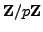The polynomials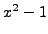has four roots in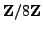, namely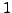,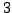,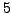, and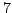. In contrast, the following proposition shows that a polynomial of degree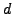over a field, such as, can have at mostroots.

Proposition 2.5 (Root Bound)   Let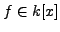be a nonzero polynomial over a field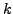. Then there are at most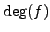elements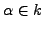such that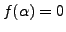.

Proof. We prove the proposition by induction on. The cases in which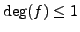are clear. Write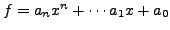. Ifthen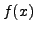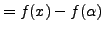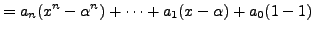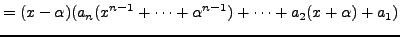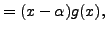for some polynomial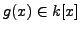. Next suppose that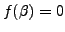with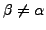. Then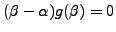, so, since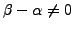andis a field, we have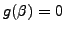. By our inductive hypothesis,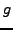has at most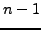roots, so there are at mostpossibilities for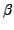. It follows that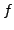has at most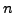roots.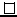SAGE Example 2.5   We use SAGE to find the roots of a polynomials over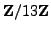.
sage: R.<x> = PolynomialRing(Integers(13))
sage: f = x^15 + 1
sage: f.roots()
[(12, 1), (10, 1), (4, 1)]
sage: f(12)
0

The output of the roots command above lists each root along with its multiplicity (which is 1 in each case above).

Proposition 2.5   Let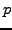be a prime number and letbe a divisor of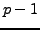. Then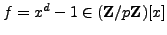has exactlyroots in.

Proof. Let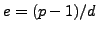. We have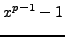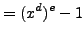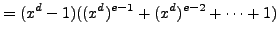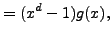where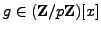and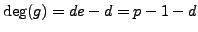. Theorem 2.1.19 implies that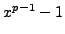has exactlyroots in, since every nonzero element ofis a root! By Proposition 2.5.3has at most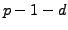roots and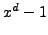has at mostroots. Since a root of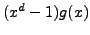is a root of eitheror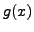andhasroots,must have exactlyroots andmust have exactlyroots, as claimed.SAGE Example 2.5   We use SAGE to illustrate the proposition.
sage: R.<x> = PolynomialRing(Integers(13))
sage: f = x^6 + 1
sage: f.roots()
[(11, 1), (8, 1), (7, 1), (6, 1), (5, 1), (2, 1)]


We pause to reemphasize that the analogue of Proposition 2.5.5 is false whenis replaced by a composite integer, since a root modof a product of two polynomials need not be a root of either factor. For example,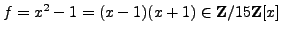has the four roots,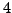,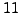, and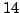.

William 2007-06-01